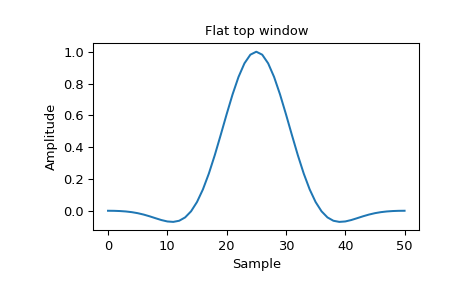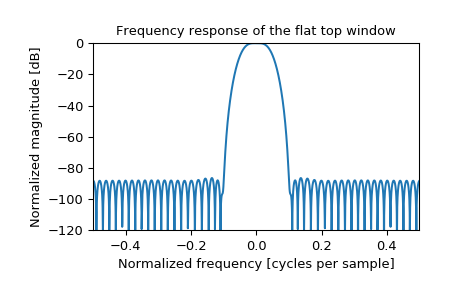# scipy.signal.windows.flattop¶

scipy.signal.windows.flattop(M, sym=True)[source]

Return a flat top window.

Parameters
Mint

Number of points in the output window. If zero or less, an empty array is returned.

symbool, optional

When True (default), generates a symmetric window, for use in filter design. When False, generates a periodic window, for use in spectral analysis.

Returns
wndarray

The window, with the maximum value normalized to 1 (though the value 1 does not appear if M is even and sym is True).

Notes

Flat top windows are used for taking accurate measurements of signal amplitude in the frequency domain, with minimal scalloping error from the center of a frequency bin to its edges, compared to others. This is a 5th-order cosine window, with the 5 terms optimized to make the main lobe maximally flat. 

References

1

D’Antona, Gabriele, and A. Ferrero, “Digital Signal Processing for Measurement Systems”, Springer Media, 2006, p. 70 DOI:10.1007/0-387-28666-7.

Examples

Plot the window and its frequency response:

>>> from scipy import signal
>>> from scipy.fft import fft, fftshift
>>> import matplotlib.pyplot as plt

>>> window = signal.flattop(51)
>>> plt.plot(window)
>>> plt.title("Flat top window")
>>> plt.ylabel("Amplitude")
>>> plt.xlabel("Sample")

>>> plt.figure()
>>> A = fft(window, 2048) / (len(window)/2.0)
>>> freq = np.linspace(-0.5, 0.5, len(A))
>>> response = 20 * np.log10(np.abs(fftshift(A / abs(A).max())))
>>> plt.plot(freq, response)
>>> plt.axis([-0.5, 0.5, -120, 0])
>>> plt.title("Frequency response of the flat top window")
>>> plt.ylabel("Normalized magnitude [dB]")
>>> plt.xlabel("Normalized frequency [cycles per sample]")